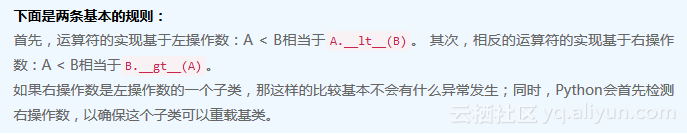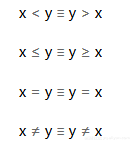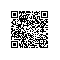# 《Python面向对象编程指南》——2.6　比较运算符方法

### 2.6　比较运算符方法

Python有6个比较运算符。这些运算符分别对应一个特殊方法的实现。根据文档，运算符和特殊方法的对应关系如下所示。

• x < y调用x.__lt__(y)。
• x <=y调用x.__le__(y)。
• x == y调用x.__eq__(y)。
• x != y调用x.__ne__(y)。
• x > y调用x.__gt__(y)。
• x >= y调用x.__ge__(y)。class BlackJackCard_p:
def __init__( self, rank, suit ):
self.rank= rank
self.suit= suit
def __lt__( self, other ):
print( "Compare {0} < {1}".format( self, other ) )
return self.rank < other.rank
def __str__( self ):
return "{rank}{suit}".format( **self.__dict__ )

>>> two = BlackJackCard_p( 2, '' )
>>> three = BlackJackCard_p( 3, '' )
>>> two < three
Compare 2 < 3
True
>>> two > three
Compare 3 < 2
False
>>> two == three
False
>>> two <= three
Traceback (most recent call last):
File "<stdin>", line 1, in <module>
TypeError: unorderable types: BlackJackCard_p() <= BlackJackCard_p()

>>> two_c = BlackJackCard_p( 2, '' )
>>>two == two_c
False@functools.total_ordering修饰符打破了这种默认行为的局限性，它可以从__eq__()或者__lt__()、__le__()、__gt__()和__ge__()的任意一个中推断出其他的比较方法。在第7章“创建数值类型”中，我们会详细探讨这种方法。

### 2.6.1　设计比较运算

• 如何比较同一个类的两个对象。
• 如何比较不同类的对象。

if hand.cards == hand.cards

if hand.cards.rank == hand.cards.rank

### 2.6.2　实现同一个类的对象比较

class BlackJackCard:
def __init__( self, rank, suit, hard, soft ):
self.rank= rank
self.suit= suit
self.hard= hard
self.soft= soft
def __lt__( self, other ):
if not isinstance( other, BlackJackCard ): return
NotImplemented
return self.rank < other.rank

def __le__( self, other ):
try:
return self.rank <= other.rank
except AttributeError:
return NotImplemented
def __gt__( self, other ):
if not isinstance( other, BlackJackCard ): return
NotImplemented
return self.rank > other.rank
def __ge__( self, other ):
if not isinstance( other, BlackJackCard ): return
NotImplemented
return self.rank >= other.rank
def __eq__( self, other ):
if not isinstance( other, BlackJackCard ): return
NotImplemented
return self.rank == other.rank and self.suit == other.suit
def __ne__( self, other ):
if not isinstance( other, BlackJackCard ): return
NotImplemented
return self.rank != other.rank and self.suit != other.suit
def __str__( self ):
return "{rank}{suit}".format( **self.__dict__)

>>> two = card21( 2, '' )
>>> three = card21( 3, '' )
>>> two_c = card21( 2, '' )

>>> two == two_c
False
>>> two.rank == two_c.rank
True
>>> two< three
True
>>> two_c < three
True

### 2.6.3　实现不同类的对象比较

>>> two = card21( 2, '' )
>>> two < 2
Traceback (most recent call last):
File "<stdin>", line 1, in <module>
TypeError: unorderable types: Number21Card() < int()
>>> two > 2
Traceback (most recent call last):
File "<stdin>", line 1, in <module>
TypeError: unorderable types: Number21Card() > int()

>>> two == 2
False
>>> two == 3
False

### 2.6.4　硬总和、软总和和多态

• 软总和把A牌当作11点。如果软总和超过21点，那么这张A牌就不可用。
• 硬总和把A牌当作1点。

• 新增一个类级别的属性。
• 新增一个方法。

def total( self ):
delta_soft = max( c.soft-c.hard for c in self.cards )
hard = sum( c.hard for c in self.cards )
if hard+delta_soft <= 21: return hard+delta_soft
return hard

### 2.6.5　不同类比较的例子

class Hand:
def __init__( self, dealer_card, *cards ):
self.dealer_card= dealer_card
self.cards= list(cards)
def __str__( self ):
return ", ".join( map(str, self.cards) )
def __repr__( self ):
return "{__class__.__name__}({dealer_card!r}, {_cards_str})".format(
__class__=self.__class__,
_cards_str=", ".join( map(repr, self.cards) ),
**self.__dict__ )

def __eq__( self, other ):
if isinstance(other,int):
return self.total() == other
try:
return (self.cards == other.cards
and self.dealer_card == other.dealer_card)
except AttributeError:
return NotImplemented
def __lt__( self, other ):
if isinstance(other,int):
return self.total() < other
try:
return self.total() < other.total()
except AttributeError:
return NotImplemented
def __le__( self, other ):
if isinstance(other,int):
return self.total() <= other
try:
return self.total() <= other.total()
except AttributeError:
return NotImplemented
__hash__ = None
def total( self ):
delta_soft = max( c.soft-c.hard for c in self.cards )
hard = sum( c.hard for c in self.cards )
if hard+delta_soft <= 21: return hard+delta_soft
return hard

>>> two = card21( 2, '' )
>>> three = card21( 3, '' )
>>> two_c = card21( 2, '' )
>>> ace = card21( 1, '' )
>>> cards = [ ace, two, two_c, three ]

>>> h= Hand( card21(10,''), *cards )
>>> print(h)
A, 2, 2, 3
>>> h.total()
18

>>> h2= Hand( card21(10,''), card21(5,''), *cards )
>>> print(h2)
5, A, 2, 2, 3
>>> h2.total()
13

>>> h < h2
False
>>> h > h2
True

>>> h == 18
True
>>> h < 19
True
>>> h > 17
Traceback (most recent call last):
File "<stdin>", line 1, in <module>
TypeError: unorderable types: Hand() > int()使用钉钉扫一扫加入圈子
+ 订阅